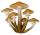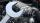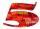# Mushrooms from the forest

Magda and Tereza goes to pick mushrooms. Total found 70 mushrooms. Magda found that between fungi found 5/9 bedel. Tereza discovered that she found among fungi are 2/17 champignons. How many mushrooms found Magda?

Result

m =  36

#### Solution:Leave us a comment of example and its solution (i.e. if it is still somewhat unclear...):Be the first to comment!#### To solve this example are needed these knowledge from mathematics:

Do you solve Diofant problems and looking for a calculator of Diofant integer equations? Do you have a linear equation or system of equations and looking for its solution? Or do you have quadratic equation?

## Next similar examples:

1. MushroomsFor five days, we have collected 410 mushrooms. Interestingly every day we have collected 10 mushrooms more than the preceding day. How many mushrooms we have collected during 4th day?
2. Theorem proveWe want to prove the sentence: If the natural number n is divisible by six, then n is divisible by three. From what assumption we started?
3. Walnutsx walnuts were in the mission. Dano took 1/4 of nuts Michael took 1/8 from the rest and John took 34 nuts. It stayed here 29 nuts. Determine the original number of nuts.
4. Mom and daughterMother is 39 years old. Her daughter is 15 years. For many years will mother be four times older than the daughter?
5. Simple equationSolve for x: 3(x + 2) = x - 18
6. Repair companyThe company repairs cars. The first day repair half of the contract second day, the half of the rest and third day 8 residue cars. How many total cars company repaired?
7. Divisible by 5How many three-digit odd numbers divisible by 5, which are in place ten's number 3?
8. Unknown numberUnknown number is divisible by exactly three different primes. When we compare these primes in ascending order, the following applies: • Difference first and second prime number is half the difference between the third and second prime numbers. • The produ
9. NumbersWrite smallest three-digit number, which in division 5 and 7 gives the rest 2.
10. Unknown numberIdentify unknown number which 1/5 is 40 greater than one tenth of that number.
11. Apples 2How many apples were originally on the tree, if the first day fell one third, the second day quarter of the rest and on tree remained 45 apples?
12. FactoryIn the factory workers work in three shifts. In the first inning operates half of all employees in the second inning and a third in the third inning 200 employees. How many employees work at the factory?
13. DivisorsThe sum of all divisors unknown odd number is 2112. Determine sum of all divisors of number which is twice of unknown numbers.
14. Fifth of the numberThe fifth of the number is by 24 less than that number. What is the number?
15. EquationSolve the equation: 1/2-2/8 = 1/10; Write the result as a decimal number.
16. CandyPeter had a sachet of candy. He wanted to share with his friends. If he gave them 30 candies, he would have 62 candies. If he gave them 40 candies, he would miss 8 candies. How many friends did Peter have?
17. Four-digit numberFind also a four-digit number, which quadrupled written backwards is the same number.